# Applied and Computational Mathematics Seminars

Upcoming Applied and Computational Mathematics Seminars
DMS Applied and Computational Mathematics Seminar
Oct 06, 2023 11:00 AM
328 Parker HallSpeaker: Habib Najm, Sandia National Laboratory

Title: TBA

Past Applied and Computational Mathematics Seminars
DMS Applied and Computational Mathematics Seminar
Sep 22, 2023 02:00 PM
328 Parker Hall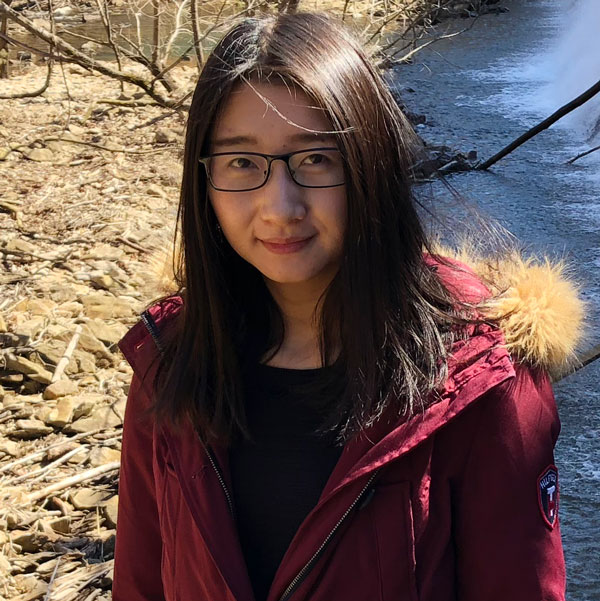Speaker: Lu Zhang (Rice University)

Title: Coupling physics-deep learning inversion

Abstract: In recent years, there has been an increasing interest in applying deep learning to geophysical/medical data inversion. However, the direct application of end-to-end data-driven approaches to inversion has quickly shown limitations in practical implementation. Indeed, due to the lack of prior knowledge of the objects of interest, the trained deep-learning neural networks very often have limited generalization. In this talk, we introduce a new methodology of coupling model-based inverse algorithms with deep learning for two typical types of inversion problems. In the first part, we present an offline-online computational strategy of coupling classical least-squares-based computational inversion with modern deep learning-based approaches for full waveform inversion to achieve advantages that cannot be achieved with only one of the components. In the second part, we present an integrated data-driven and model-based iterative reconstruction framework for joint inversion problems. The proposed method couples the supplementary data with the partial differential equation model to make the data-driven modeling process consistent with the model-based reconstruction procedure. We also characterize the impact of learning uncertainty on the joint inversion results for one typical inverse problem.

DMS Applied and Computational Mathematics Seminar
Sep 15, 2023 02:00 PM
328 Parker Hall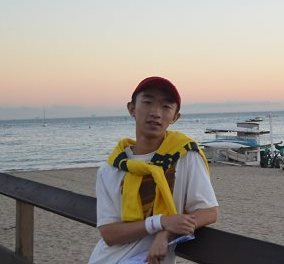Speaker: Yuming Paul Zhang, Auburn University

Title: Exploratory HJB Equations and Their Convergence

Abstract: We study the exploratory Hamilton--Jacobi--Bellman (HJB) equation arising from the entropy-regularized exploratory control problem, which was formulated by Wang, Zariphopoulou, and Zhou (J. Mach. Learn. Res., 21, 2020) in the context of reinforcement learning in continuous time and space. We establish the well-posedness and regularity of viscosity solutions to the equation, and derive an explicit rate of convergence as exploration diminishes to zero. If time permits, I would also discuss the analysis of the policy iteration algorithm used to study the control problem. These are joint works with Xunyu Zhou, Hung Tran, and Wenpin Tang.

DMS Applied and Computational Mathematics Seminar
Sep 08, 2023 02:00 PM
328 Parker Hall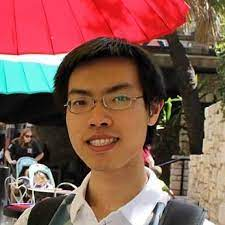Speaker: Yimin Zhong, Auburn University

Title: Implicit boundary integral method for linearized Poisson Boltzmann equation

Abstract: In this talk, I will give an introduction to the so-called implicit boundary integral method based on the co-area formula and it provides a simple quadrature rule for boundary integral on general surfaces.  Then, I will focus on the application of solving the linearized Poisson Boltzmann equation, which is used to model the electric potential of protein molecules in a solvent. Near the singularity, I will briefly discuss the choices of regularization/correction and illustrate the effect of both cases. In the end, I will show the numerical error estimate based on the harmonic analysis tools.

DMS Applied and Computational Math
Sep 01, 2023 02:00 PM
328 Parker HallSpeaker: Cao Kha Doan (Auburn University)

Title: Low regularity integrators for the classical and conservative Allen-Cahn equations with maximum bound principles

Abstract: This talk is concerned with conditionally structure-preserving, low regularity time integration methods for both classical and conservative Allen–Cahn equations. Important properties of such equations include the maximum bound principle (MBP) and energy dissipation law. By iteratively using Duhamel’s formula, first- and second-order low regularity integrators (LRIs) are constructed for time discretization. The proposed LRI schemes are proved to preserve the MBP and energy stability, and are shown to conserve mass for the conservative Allen-Cahn equation. Furthermore, their temporal error estimates are also successfully derived under a low regularity requirement that the exact solution is only assumed to be continuous in time. Numerical results show that the proposed LRI schemes are more accurate and have better convergence rates than the exponential time differencing schemes, especially when the interfacial parameter approaches zero.

Applied and Computational Mathematics
Apr 28, 2023 02:00 PM
328 Parker Hall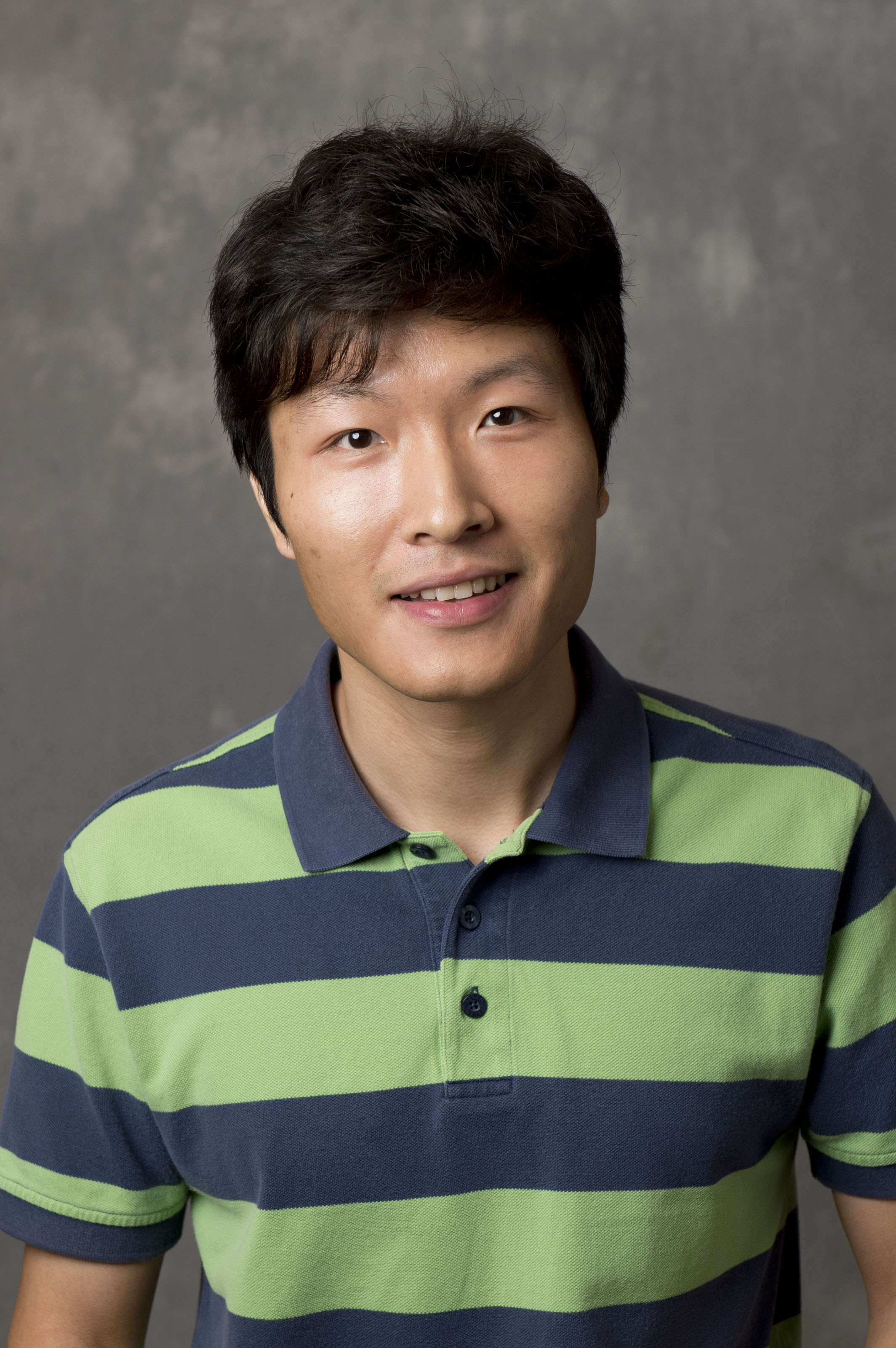Speaker: Yiran Wang, Emory University

Title: Analysis and reduction of metal artifacts in X-ray tomography

Abstract: Due to beam-hardening effects, metal objects in X-ray CT often produce streaking artifacts which cause degradation in image reconstruction. It is known from the work of Seo et al in 2017 that the nature of the phenomena is nonlinear. An outstanding inverse problem is to identify the nonlinearity which is crucial for reduction of the artifacts. In this talk, we show how to use microlocal techniques to analyze the artifacts and extract information of the nonlinearity. In particular, we discuss the interesting connection between the artifact generation and geometry of metal objects.

DMS Applied and Computational Mathematics
Apr 21, 2023 02:00 PM
ZOOMSpeaker:  Assistant Professor Peng Chen, Georgia Tech

Title: Scalable Bayesian optimal experimental design for efficient data acquisition

Abstract: Bayesian optimal experimental design (OED) is a principled framework for maximizing information gained from limited data in Bayesian inverse problems. Unfortunately, conventional methods for OED are prohibitive when applied to expensive models with high-dimensional parameters. In this talk, I will present fast and scalable computational methods for large-scale Bayesian OED with infinite-dimensional parameters, including data-informed low-rank approximation, efficient offline-online decomposition, projected neural network approximation, and a new swapping greedy algorithm for combinatorial optimization.

DMS Special Seminar in Analysis
Apr 17, 2023 02:00 PM
250 Parker Hall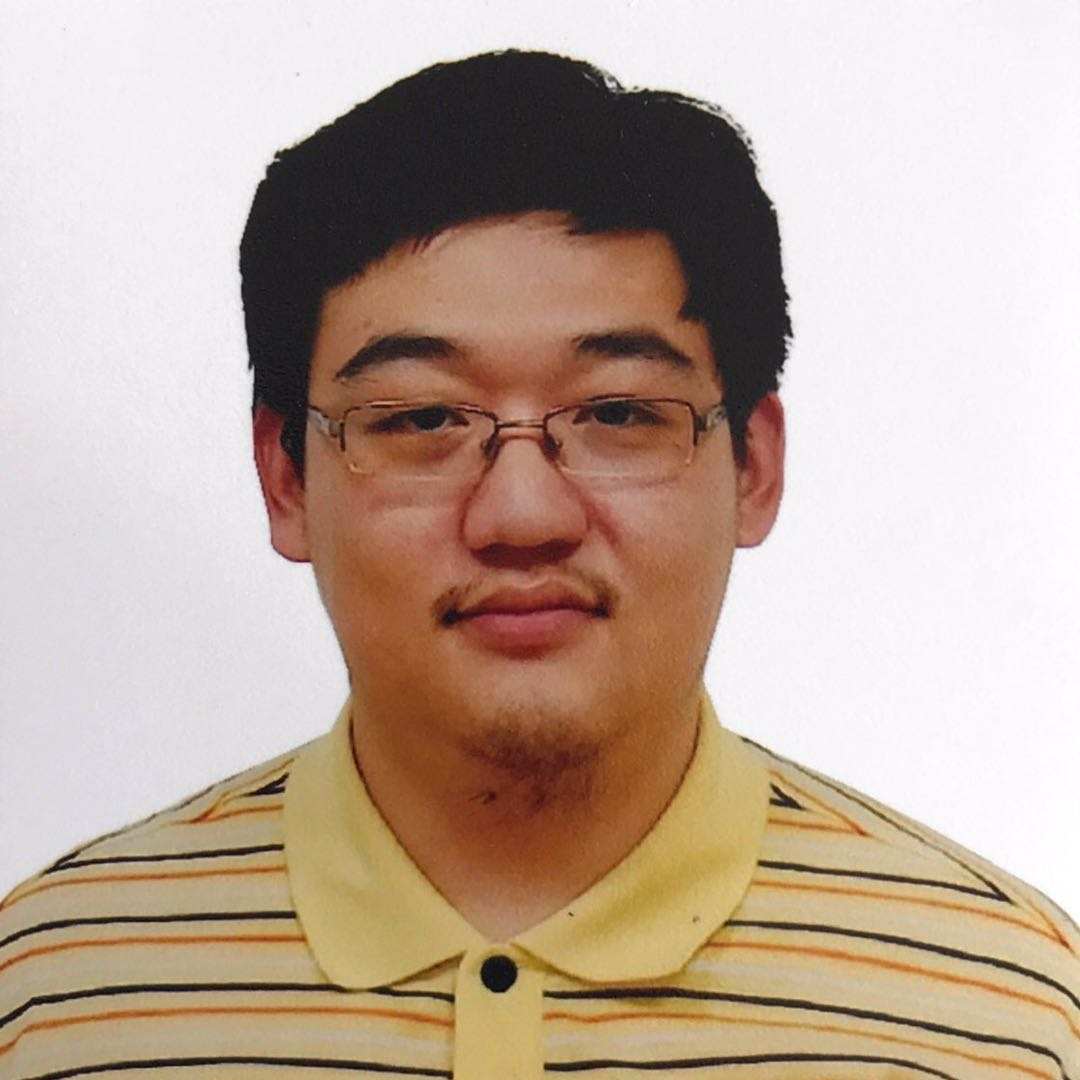Speaker: is Dr. Bingyang Hu, Purdue University

Title: Suppression of Epitaxial Thin Film Growth by Mixing

Abstract: In this talk, we consider the fourth-order parabolic equation with gradient nonlinearity on the two-dimensional torus, with or without an advection term. We will show that in the absence of advection, there exists initial data which will make the solution blow up in finite time; while in the advective case, if the imposed advection is sufficiently mixing, the global existence of the solutions can be achieved. Finally, we will make some further remarks on the general framework on how advection can guarantee the global existence of certain non-linear equations.

This talk is based on several joint works with Yu Feng, Xiaoqian Xu, and Yeyu Zhang.

DMS Applied and Computational Mathematics
Apr 14, 2023 02:00 PM
328 Parker HallSpeaker: Evdokiya (Eva) Kostadinova, Auburn University, Department of Physics

Title: Fractional Laplacian Spectral Approach (FLS) to Anomalous Diffusion of Energetic Particles in Magnetized Plasma

Abstract: Fractional-power operators have received much attention due to their wide application in modeling anomalous diffusion. For the fractional Laplacian $$(−Δ)^{\it s}$$, values $$𝑠∈(0,1)$$ correspond to a superdiffusive process, while $$𝑠∈(1,2)$$ defines the subdiffusion regime. Physically, subdiffusion can be thought of as superposition of classical diffusion and a small superdiffusive part. Such a process results in overall trapping of the particle ensemble, while also allowing for nonlocal jumps. It can also be shown that for $$𝑠∈(3/2,2)$$, the transport process is bounded leading to probability distribution functions with truncated tails. We also argue that in the superdiffusive regime, for $$𝑠∈(2/3,1)$$, particles exhibit nonlocal jumps, but transport is described by a true Lévy process only for values $$𝑠∈(0,2/3)$$. Thus, we expect that there are at least four regimes of anomalous diffusion: strong trapping, trapping with nonlocal jumps, nonlocal jumps without trapping, and Lévy flights.

To investigate the proposed sub-regimes of anomalous diffusion, we use a Fractional Laplacian Spectral (FLS) method, where the existence of extended states (interpreted as probability for transport) is determined from the existence of a continuous part in the spectrum of a Hamiltonian. Here we are interested in a Hamiltonian with a fractional Laplacian term and a stochastic disorder term. The range of nonlocal interactions and the amount of stochasticity for the Hamiltonian are informed from experiments where energetic electrons (EEs) were detected in the presence of magnetic islands and stochastic magnetic fields. The spectral properties of each Hamiltonian are investigated for fractions representative of each diffusion regime. Comparison of these calculations to experimental data reveals the presence of at least two types of EEs: runaway electrons, best described by a Lévy process, and suprathermal, but non-relativistic, electrons, best described by trapping with nonlocal jumps.

This is joint work with B. Andrew and D. M. Orlov.

Work supported by DE-FC02-04ER54698, DE- FG02-05ER54809, and DE-SC0023061.

DMS Applied and Computational Mathematics
Apr 14, 2023 11:00 AM
328 Parker Hall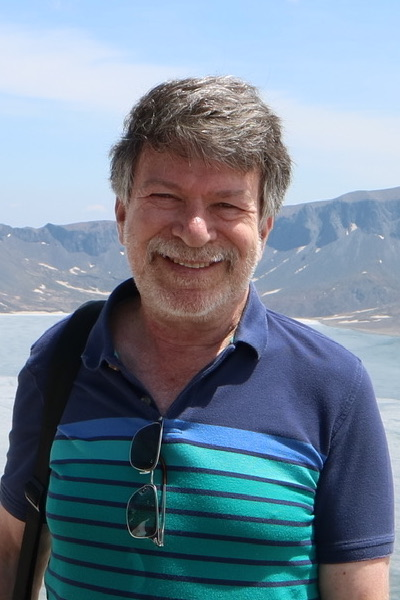Speaker: Amnon J Meir, Southern Methodist University

Title: On the Equations of Electroporoelasticity

Abstract: Complex physical phenomena and systems frequently involve multiple components, complex physics or multi-physics, as well as complex or coupled domains and multiple scales. Such phenomena are usually modeled by systems of coupled partial differential equations, often nonlinear. One such phenomenon is electroporoelasticity.

After introducing the equations of electroporoelasticity (the equations of poroelasticity coupled to Maxwell's equations) which have applications in geoscience, hydrology, and petroleum exploration, as well as various areas of science and technology, I will describe some recent results (well posedness), the numerical analysis of a finite-element based method for approximating solutions, and some interesting challenges.

DMS Applied and Computational Mathematics
Apr 07, 2023 02:00 PM
328 Parker Hall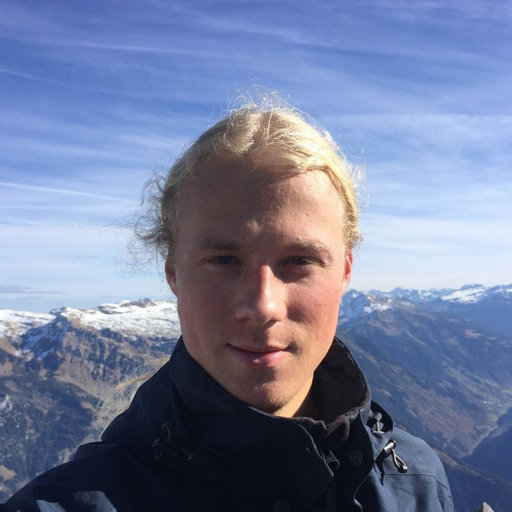Speaker: Erik Hiltunen, Yale University

Title: Scattering phenomena and spectral convergence of subwavelength resonators

Abstract: We study wave propagation inside metamaterials consisting of high-contrast subwavelength resonators. In the subwavelength limit, resonant states are described by the eigenstates of the generalized capacitance matrix, which describes a long-range, fully-coupled resonator model. We achieve this by re-framing the Helmholtz equation as a non-linear eigenvalue problem in terms of integral operators. In this setting, we survey a range of subwavelength resonance phenomena such as Anderson localization, topologically protected edge modes, exceptional points, and Fano resonance. Additionally, we discuss the spectral convergence of finite structures. As the size of the structure increases, we show that defect modes induced by compact perturbations converge to localized modes of the infinite structure. Using Toeplitz eigenvalue distribution results, we additionally demonstrate the distributional convergence of the density of states as the size of the structure increases.

Short Bio: Dr. Erik O. Hiltunen is a Gibbs Assistant Professor at Yale University, USA. Dr. Hiltunen's research focuses on developing the mathematical understanding of wave propagation in materials governed by local or non-local PDEs, using tools from PDE theory, harmonic analysis and solid-state physics. Before moving to Yale, Hiltunen earned his PhD from ETH Zurich under the supervision of prof. Habib Ammari, where his thesis dissertation was awarded the ECCOMAS award for best PhD theses on Computational Methods in Applied Sciences.